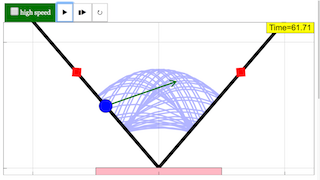IntroPage

# Ball in Wedge

Ball in Wedge models the dynamics of a ball falling and bouncing elastically in a symmetric wedge. Although the ball's free-fall trajectory and bounce are simple and are discussed in introductory physics textbooks, the ball-wedge system exhibits a rich array of periodic and chaotic behavior and is of current research interest.

The ball has both chaotic and periodic trajectories but it is difficult to find initial conditions (x0, vx0, y0, vy0) that lead to periodicity. The "trick" is to examine the dynamics when the ball collides with the wall. At the time of collision, we record the ball's velocity component tangent to the wall vr and its velocity component perpendicular to the wall vn. A plot of vr vs. vn2 at the time of impact, known as a Poincaré section, provides a stroboscopic snapshot of the ball's state. The measured velocity components determine the ball's position because we can compute the ball's kinetic energy and use conservation of energy to compute the ball's height. The x-coordinate is computed using the y-coordinate and the wedge angle.

Run the Ball in Wedge model and observe the patterns. If the motion is periodic, the snapshot (Poincaré section) will show only a small number of points corresponding the number of collisions within the periodic orbit. If the trajectory is chaotic there the points on the plot will appear to be random. Initial conditions are selected by dragging the ball or by clicking within the Poincaré plot. Notice the symmetry of the patterns. Clicking at the center of an oval reveals the periodic trajectory.

### Translations

Code Language Translator Run### Software Requirements

SoftwareRequirements

 Android iOS Windows MacOS with best with Chrome Chrome Chrome Chrome support full-screen? Yes. Chrome/Opera No. Firefox/ Samsung Internet Not yet Yes Yes cannot work on some mobile browser that don't understand JavaScript such as..... cannot work on Internet Explorer 9 and below

### CreditsThis email address is being protected from spambots. You need JavaScript enabled to view it. - WEH-Foundation; Fremont Teng; Loo Kang Wee; Francisco Esquembre

[text]

## Ball in Wedge JavaScript Simulation Applet HTML5

Ball in Wedge models the dynamics of a ball falling and bouncing elastically in a symmetric wedge. Although the ball's free-fall trajectory and bounce are simple and are discussed in introductory physics textbooks, the ball-wedge system exhibits a rich array of periodic and chaotic behavior and is of current research interest.

The ball has both chaotic and periodic trajectories but it is difficult to find initial conditions (x0, vx0, y0, vy0) that lead to periodicity. The "trick" is to examine the dynamics when the ball collides with the wall. At the time of collision, we record the ball's velocity component tangent to the wall vr and its velocity component perpendicular to the wall vn. A plot of vr vs. vn2 at the time of impact, known as a Poincaré section, provides a stroboscopic snapshot of the ball's state. The measured velocity components determine the ball's position because we can compute the ball's kinetic energy and use conservation of energy to compute the ball's height. The x-coordinate is computed using the y-coordinate and the wedge angle.

Run the Ball in Wedge model and observe the patterns. If the motion is periodic, the snapshot (Poincaré section) will show only a small number of points corresponding the number of collisions within the periodic orbit. If the trajectory is chaotic there the points on the plot will appear to be random. Initial conditions are selected by dragging the ball or by clicking within the Poincaré plot. Notice the symmetry of the patterns. Clicking at the center of an oval reveals the periodic trajectory.

### Instructions

#### High Speed Check Box

Checking this will set the speed of the simulation to be fast or slow.

Drag the two red boxes to adjust the angle of the two wedges.

(Default)

(Pulling wedges outwards)

Notices that the trails of the particle will differ based on the angle between the two wedges.

#### Toggling Full Screen

Double clicking inside the panel will toggle full screen.
Note that this won't work if the simulation is playing.

#### Play/Pause, Step and Reset Buttons

Plays/Pauses, steps and resets the simulation respectively.

Research

[text]

[text]

[text]

### end faqRating 0.00 (0 Votes)